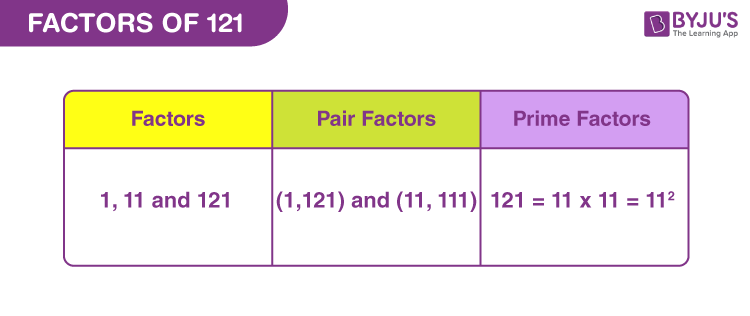# Factors of 121

Factors of 121 are the real numbers that divide the original number, completely. When we divide 121 by one of its factors, then the remainder is zero and quotient is a whole number. For example, 3 is the factor of 9 because when we divide 9 by 3 we get;

9 divided by 3 ⇒ 9 ÷ 3 = 3

Thus, we get a whole number as the quotient after division.

We can also say, if the product of two numbers results in 121, then the two numbers are the factors of 121. Prime factor form of 121 is the product of primes that results in the original number.## How to Find Factors of 121?

Factors of 121 are the numbers that divide the original number, without leaving any remainder. We will start dividing 121 by the smallest natural number 1. As we know, 121 is an odd number, therefore, it cannot be divided by any even number.

• 121 ÷ 1 = 121
• 121 ÷ 11 = 11
• 121 ÷ 121 = 1

If we divide 121 by any other number, then the quotient will be a fraction and not a whole number. For example, 121 divided by 10 is 12.1.

Thus, we can conclude that only 1, 11 and 121 are the factors of 121.

## Pair Factors of 121

To find the pair factors of 121, we need to find the product of the two numbers, to get the original number.

• 1 × 121 = 121
• 11 × 11 = 121

Therefore, the pair factors are (1, 121) and (11, 11). As we can see, these are the positive pair factors. Similarly, if we consider negative pair factors of 121, then the multiplication of such two negative factors will result in a positive value.

• -1 × -121 = 121
• -11 × -11 = 121

Therefore, the negative pair factors are (-1, -121) and (-11, -11).

## Prime Factorisation of 121

To find the prime factors of 121 we need to divide 121, starting with the smallest prime number, till the remainder is 1. Since 121 is directly divisible by 11 and no other prime factors are less than 11, thus;

Step 1: Dividing 121 by the smallest prime factor, which is 11, we get;

121/11 = 11

Step 2: Again divide 11 by the smallest prime factor, 11, to get;

11/11 = 1

Step 3: Now, it is not possible to divide 1 any further. Therefore,

 Prime factorisation of 121 = 11 x 11

## Solved Examples

Q.1: If there are 121 pens to be distributed among 11 students in a class. How many pens does each student get?

Solution: Given,

Number of pens = 121

Number of students in class = 11

Each student will get = 121/11 = 11 pens

Q.2: What is the sum of all the factors of 121?

Solution: The factors of 121 are 1, 11 and 121.

Sum = 1+11+121 = 133

Therefore, 133 is the required sum.

Q.3: What are the common factors of 101 and 121?

Answer: To find the common factors of 101 and 121, we need to find the factors of each of the given numbers.

101 is a prime number, therefore, it has only two factors.

101 → 1, 101

But 121 is the composite number, therefore factors of:

121 → 1, 11 and 121

Hence, we can see, the common factor is only 1.

## Frequently Asked Questions on Factors of 121

### 1. How many factors of 121 are there?

There are three factors of 121. They are 1, 11 and 121.

### 2. Are the multiples and factors of 121 the same?

No. Multiples and factors are different for all the natural numbers. There are only three factors for 121 but it has indefinitely many multiples.

### 3. What are the multiples of 121?

The first 10 multiples of 121 are 1121, 242, 363, 484, 605, 726, 847, 968, 1089 and 1210.

### 4. What are the prime factors of 121?

The prime factorisation of 121 is equal 11 x 11 = 112. Therefore, 11 is the prime factor of 121.

### 5. What is the average value of factors of one hundred and twenty-one?

Answer: Average value is the sum of all the values divided by the total number of values

Average (factors of 121) = (1+11+121)/3 = 133/3